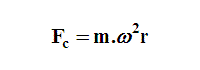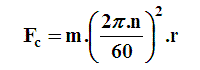Science

Dynamics

 Show figure Calculate:   Centrifugal Force (Fc) Mass (m) Centripetal Acceleration (ac) Tangential Velocity (Vt) Angular Velocity (w) Rotational Speed (n) Fc=   N m =   kg ac=   m/s2 Vt =   m/s r =   m w =   rad/s n =   rpmTo Memory 01 Memory 02 Memory 03 Memory 04 Memory 05 Memory 06 Replacing Adding Subtracting Multiplying Dividing
 Configurations Maximum of decimal places Units:   MKS (SI)    MK*S    CGS    English Units Impression Page Title (Optional) Comments to be printed (Optional)